### Direct Covariance Structures Analysis

Previous examples are concerned with the implied covariance structures from the functional relationships among manifest and latent variables. In some cases, direct modeling of the covariance structures is not only possible, but indeed more convenient. The MSTRUCT modeling language in PROC CALIS is designed for this purpose. Consider the following four variables from the Wheaton et al. (1977) data:

Anomie67:

Anomie 1967

Powerless67:

Powerlessness 1967

Anomie71:

Anomie 1971

Powerless71:

Powerlessness 1971

The covariance structures are hypothesized as follows: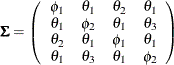where: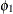:

Variance of Anomie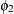:

Variance of Powerlessness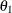:

Covariance between Anomie and Powerlessness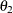:

Covariance between Anomie measures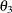:

Covariance between Powerlessness measures

In the hypothesized covariance structures, the variances of Anomie and Powerlessness measures are assumed to stay constant over the two time points. Their covariances are also independent of the time of measurements. To test the tenability of this covariance structure model, you can use the following statements of the MSTRUCT modeling language:

proc calis nobs=932 data=Wheaton;
mstruct
var = Anomie67 Powerless67 Anomie71 Powerless71;
matrix _COV_ [1,1] = phi1,
[2,2] = phi2,
[3,3] = phi1,
[4,4] = phi2,
[2,1] = theta1,
[3,1] = theta2,
[3,2] = theta1,
[4,1] = theta1,
[4,2] = theta3,
[4,3] = theta1;
run;


In the MSTRUCT statement, you specify the list of variables of interest with the VAR= option. The order of the variables in the list will be the order in the hypothesized covariance matrix. Next, you use the MATRIX _COV_ statement to specify the parameters in the covariance matrix. The specification is a direct translation from the hypothesized covariance matrix. For example, the [1,1] element of the covariance matrix is fitted by the free parameter phi1. Depending on the hypothesized model, you can also specify fixed constants for the elements in the covariance matrix. If an element in the covariance matrix is not specified by either a parameter name or a constant, it is assumed to be a fixed zero.

The analysis of this model is carried out in Example 27.19.

The MSTRUCT modeling language appears to be more restrictive than any of the other modeling languages discussed, in regard to the following limitations:

• It does not explicitly support latent variables in modeling.

• It does not explicitly support modeling of linear functional relations among variables (for example, paths).

However, these limitations are more apparent than real. In PROC CALIS, the parameters defined in models can be dependent. These dependent parameters can be defined further as functions of other parameters in the PARAMETERS and the SAS programming statements. With these capabilities, it is possible to fit structural models with latent variables and with linear functional relations by using the MSTRUCT modeling language. However, this requires a certain level of sophistication in statistical knowledge and in programming. Therefore, it is recommended that the MSTRUCT modeling language be used only when the covariance and mean structures are modeled directly.

For more information about the MSTRUCT modeling language, see the section The MSTRUCT Model and the .